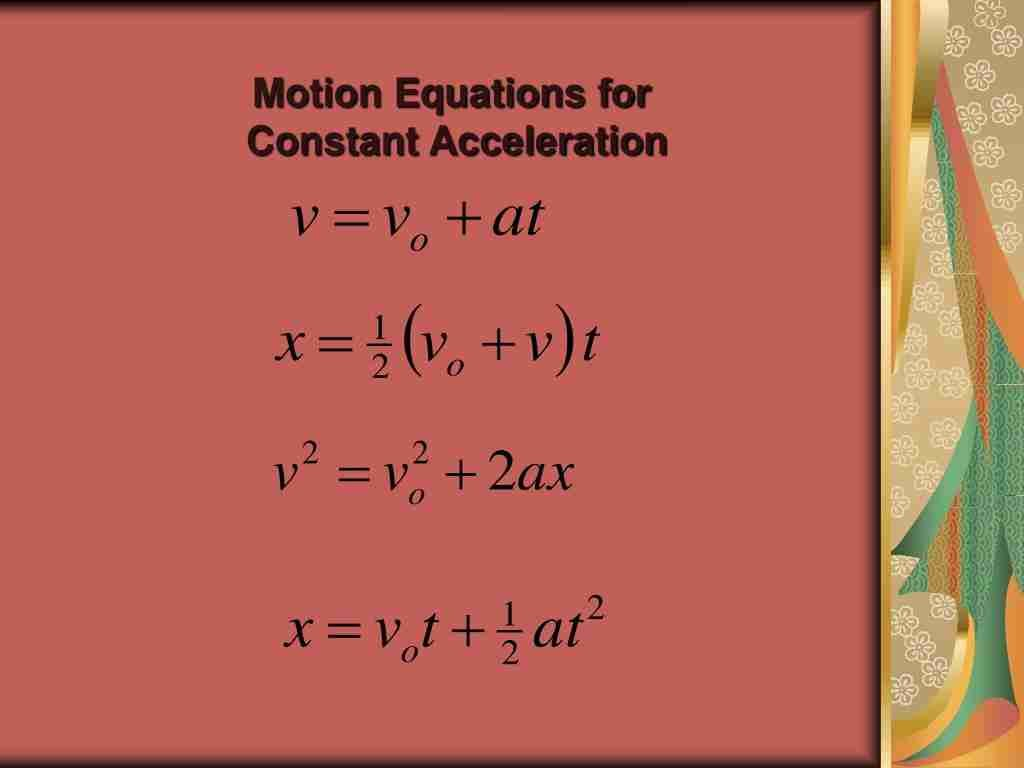How Cheenta works to ensure student success?
Explore the Back-Story

# NSEP 2015 Problem 8 | One Dimensional MotionTry out this problem on one dimensional motion(Kinematics) from National Standard Examination in Physics 2015-2016.

## NSEP 2015-16 ~ Problem 8

A car moving along a straight road at a speed of u m/s applies brakes at $t=0$ seconds. The ratio of distances travelled by the car during $3^{rd}$ and $8^{th}$ seconds is $15:13$. The car covers a distance of $0.25m$ in the last second of it's travel. Therefore, the acceleration a (in $m/s^2$) and the speeed u($m/s$) of the car are respectively,

a) $-0.1,16$

b) $-0.2,12$

c) $-0.5,20$

d) $-0.1,16$

### Key Concepts

Basic Equation of motions

Idea of accelerations, velocity and displacement

## Suggested Book | Source | Answer

Concept of Physics H.C. Verma

University Physics by H. D. Young and R.A. Freedman

Fundamental of Physics D. Halliday, J. Walker and R. Resnick

National Standard Examination in Physics(NSEP) 2015-2016

Option-(c) $-0.5,20$

## Try with Hints

We know that displacement in $t$ sec is given by,

$$s = ut +\frac{1}{2} a t^2$$

where $u$ is the initial velocity and $a$ is the acceleration. This will be negative for deceleration.

Using this idea we can just find the expression for the displacement in the $n^{th}$ second, for which the information is given to us.

Also, the car come to rest and at the second second it travels a distance of $0.25m$. WE can think it in opposite manner that with an acceleration of $a$ and initial velocity $0m/s$, the car moves a distance of $0.25m$ , hence,

$$0.5 =\frac{1}{2} a (1^2)$$

So, the displacement in $n^{th}$ second is,

$$s_1 = ut+\frac{1}{2} a n^2$$

and for the $(n-1)^{th}$ second the displacement is,

$$s_2= ut+\frac{1}{2} a (n-1)^2$$

Hence, we have

$$s_n = s_1-s_2 = u + a(n-\frac{1}{2})$$

we have,

$$\frac{15}{13} = \frac{s_3}{s_8} = \frac{u-\frac{5a}{2}}{u - \frac{15a}{2}}$$

Also we have $0.5 =\frac{1}{2} a (1^2)$

Solving this two gives us $a = 0.25m/s^2$. Putting this in first equation gives us $u = 20 m/s$.

## Subscribe to Cheenta at Youtube

Try out this problem on one dimensional motion(Kinematics) from National Standard Examination in Physics 2015-2016.

## NSEP 2015-16 ~ Problem 8

A car moving along a straight road at a speed of u m/s applies brakes at $t=0$ seconds. The ratio of distances travelled by the car during $3^{rd}$ and $8^{th}$ seconds is $15:13$. The car covers a distance of $0.25m$ in the last second of it's travel. Therefore, the acceleration a (in $m/s^2$) and the speeed u($m/s$) of the car are respectively,

a) $-0.1,16$

b) $-0.2,12$

c) $-0.5,20$

d) $-0.1,16$

### Key Concepts

Basic Equation of motions

Idea of accelerations, velocity and displacement

## Suggested Book | Source | Answer

Concept of Physics H.C. Verma

University Physics by H. D. Young and R.A. Freedman

Fundamental of Physics D. Halliday, J. Walker and R. Resnick

National Standard Examination in Physics(NSEP) 2015-2016

Option-(c) $-0.5,20$

## Try with Hints

We know that displacement in $t$ sec is given by,

$$s = ut +\frac{1}{2} a t^2$$

where $u$ is the initial velocity and $a$ is the acceleration. This will be negative for deceleration.

Using this idea we can just find the expression for the displacement in the $n^{th}$ second, for which the information is given to us.

Also, the car come to rest and at the second second it travels a distance of $0.25m$. WE can think it in opposite manner that with an acceleration of $a$ and initial velocity $0m/s$, the car moves a distance of $0.25m$ , hence,

$$0.5 =\frac{1}{2} a (1^2)$$

So, the displacement in $n^{th}$ second is,

$$s_1 = ut+\frac{1}{2} a n^2$$

and for the $(n-1)^{th}$ second the displacement is,

$$s_2= ut+\frac{1}{2} a (n-1)^2$$

Hence, we have

$$s_n = s_1-s_2 = u + a(n-\frac{1}{2})$$

we have,

$$\frac{15}{13} = \frac{s_3}{s_8} = \frac{u-\frac{5a}{2}}{u - \frac{15a}{2}}$$

Also we have $0.5 =\frac{1}{2} a (1^2)$

Solving this two gives us $a = 0.25m/s^2$. Putting this in first equation gives us $u = 20 m/s$.

## Subscribe to Cheenta at Youtube

This site uses Akismet to reduce spam. Learn how your comment data is processed.

### Knowledge Partner